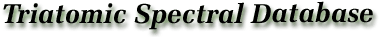4. List of Symbols

a. Quantum Numbers

 J Resultant total angular momentum quantum number, excluding nuclear spins. N Rotational angular momentum quantum number, excluding electron and nuclear spins, in the case where electron spin is present. K Projection of J (or N) on the symmetry axis in the limiting prolate or oblate symmetric top. F1 Resultant angular momentum quantum number including nuclear spin for one nucleus. F Resultant total angular momentum quantum number. λ or ε Quantum number employed when F1 is not a good quantum number. This value simply numbers the levels from lowest to highest energy for the same F quantum number. ν1,ν2,ν3 υ1 υ2 υ3 Vibrational modes (ν) and quantum numbers (υ). ν1 is the highest energy symmetric stretching mode, ν2 is the bending mode, and ν3 is the asymmetric stretch for XY2 molecules or lowest energy stretch for XYZ molecules. U or L Upper or lower energy level or transition frequency. ′ or ″ Prime or double prime is used to distinguish the upper (′) and lower (″) levels in a transition. They occur as superscripts on the quantum numbers. ℓ Quantum number for vibrational angular momentum. I or (Ii ) Angular momentum quantum number of nuclear spin for one (or ith) nucleus. S Resultant angular momentum number of electron spins. Σ is the projection of S on the molecular axis. Λ Absolute values of the projection of the resultant orbital angular momentum on the molecular axis. Ω Absolute value of the projection of the total electronic angular momentum on the molecular axis. Σ, Π, Δ Electronic state designation for which Λ = 0,1,2, respectively.

b. Molecular Constants

A, B, C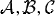Rotational constants (MHz). These are related to the principal moments of inertia: A = h/8π2 Ia, etc.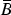B-bar equals (B + C)/2.
τ, Δ, δ, D Quartic centrifugal distortion constants (MHz or kHz).
H, h Sextic centrifugal distortion constants (MHz or kHz).
L, , G, g Octic centrifugal distortion constants (MHz).
P, p, F Dectic centrifugal distortion constants (MHz).
eQqaa(X), ...
χaa(X), ...
Nuclear electric quadrupole coupling constant along principal axis indicated for nucleus X (MHz).
eQq η Product of the nuclear quadrupole coupling constant and the asymmetry parameter for the bending vibrational state.
µa,b Components of the electric dipole moment along the a- or b-principal axes.
αυ , γυυ Rotation-vibration coefficients in the power series representing Bυ (eq 3).
qυ -doubling constant (MHz).
σ|| , σ Components of the magnetic shielding tensor which are parallel and perpendicular to the molecular axis, respectively.
Q Molecular quadrupole moment relative to the center of mass (esu · cm2).
α|| - α Electric polarizability anisotropy (cm3).
χ χ|| Components of the magnetic susceptibility tensor which are, respectively, perpendicular and parallel to the molecular axis (erg/G2 · mol).
χxx - χyy Magnetic susceptibility anisotropy.
g , g|| Components of the molecular G tensor which are, respectively, perpendicular and parallel to the molecular axis. g is sometimes denoted g or gJ for linear molecules in the ground state (µN).
gxx - gyy Anisotropy of the molecular G tensor perpendicular to the molecular axis (µN).
cX or M Spin rotation constant related to nucleus X (kHz).
SXY Spin-spin interaction constant between nucleus X and nucleus Y (kHz).
αp , βp Ω-type doubling parameters,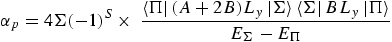(eq14)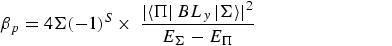(eq15)

peff Λ-type doubling constant in the 2Π1/2 state (MHz).
a, b, c, d Magnetic hyperfine coupling constants (MHz) where,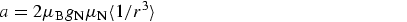(eq16)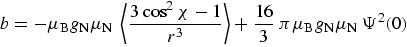(eq17)(eq18)(eq19)

Here µB is the Bohr magneton, µN is the nuclear magneton, and gN is the nuclear g-value.

A Spin-orbit coupling constants defined by the power series expansion, A = Ae + A(1) ξ + A(2) ξ2 + ... .
(O)S Spin-rotation interaction constant (ref ). Coefficient in the Hamiltonian term (O)S N · S. This is related to εii's in Lin's notation  as (O)S = 1/3(εxx + εyy + εzz).
(ij)S,Tij Spin-rotation interaction constants where i and j are a, b, or c and the Hamiltonian term is Σ(ij)S NiSj. See Curl and Kinsey  and Bowater et al.  (for Tij notation) for correspondence to the notation of other workers.
(O)I,aI Fermi interaction constant and coefficient of the I · S operator. (O)I = (16π/3)gI µB µN [Ψ(O)]2.
(ij)I Magnetic dipole-dipole interaction constant in the Hamiltonian term (ij)I SiIj where i and j are a, b, or c. (ij)S = gS gI µB µN [δij - (3rirj / r3]Av .
(ij)Q Nuclear electric quadrupole interaction constant in the term (ij)Q IiIj where i and j are a, b, or c and (ij)Q = [eQ / 2I(2I - l)] (∂2V / ∂ij).
AS , BS , CS Combination of spin-rotation constants where AS = (aa)S + (O)I , BS = (bb)S + (O)I , and CS = (cc)S + (O)I .

c. Other

 * Asterisks in the uncertainty column indicate that the transition frequency is calculated rather than measured. (...) Parentheses in the numerical listings contain measured or estimated uncertainties. For example, the value 1.407(83) should be interpreted as 1.407 ± 0.083. Thus the value in parentheses refers to the last significant digits given. a,b,c Designate principal axes corresponding to A, B, and C, respectively. r(X-Y) Distance between centers of mass of atoms X and Y (Å). ∠XYZ Angle formed by atoms X, Y, and Z (degrees).